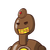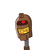# In a flight of 600 kms, an aircraft was slowed down due to bad weather and the average speed was reduced by 200 km/hour and the ti

In a flight of 600 kms, an aircraft was slowed down due to bad weather and the average speed was reduced by 200 km/hour and the time of flight increased by 30 minutes. What is the total duration of flight?

Kindly don’t spam ! ​

### 2 thoughts on “In a flight of 600 kms, an aircraft was slowed down due to bad weather and the average speed was reduced by 200 km/hour and the ti”

1.Given :

• Distance Covered by flight =600kms
• Speed reduced by a flight = 200km/hour
• Time increased by a flight = 30 min (1/2 hours)

To Find

• Total duration of the flight.

Formula Applied :

• We have to calculate the total duration occured by the flight, and it’s given that the flight of distance 600kms reduced the speed by 200km/hour and increased time by 30 minutes. Hence the Reduced Speed can be calculated by reducing the New speed from the original speed of the flight.

$$\underline{\boxed{\sf Reduced_{(Speed)}=Original_{(Speed)}-New_{(Speed)}}}$$

• For applying this formula, first we have to know the Speed formula as given as below :-

$$\underline{\boxed{\sf Speed = \dfrac{Distance}{Time \:taken}}}$$

Solution :

Let the total duration covered by the flight be x.

formula to be used,

$$\boxed{\sf Reduced_{(Speed)} =Original_{(Speed)}-New_{(Speed)}}$$

• Reduced Speed = 200km/hour
• Distance = 600kms
• Original duration = x
• New duration = $$\sf x+\frac{1}{2}$$

Substituting values in formula,

$${\Rightarrow \sf 200 = \dfrac{600}{x}-\dfrac{600}{x+\dfrac{1}{2}}}\\$$

$${\Rightarrow \sf 200=\dfrac{600}{x}-\dfrac{600}{\dfrac{2x+1}{2}}}\\$$

$${\Rightarrow \sf 200 = \dfrac{600}{x}-\dfrac{600\times 2}{2x+1}}\\$$

$${\Rightarrow \sf 200=\dfrac{600}{x}-\dfrac{1200}{2x+1} }\\$$

$${\Rightarrow \sf 200 = \dfrac{(2x+1)(600)-(1200)(x)}{(x)(2x+1)} }\\$$

$${\Rightarrow \sf 200=\dfrac{(1200x+600)-(1200x)}{2x^2+x}}\\$$

$${\Rightarrow \sf 200 = \dfrac{{\cancel{1200x}}+600-{\cancel{1200x}}}{2x^2+x}}\\$$

$${\Rightarrow \sf 200 = \dfrac{600}{2x^2+x}}\\$$

$${\Rightarrow \sf 2x^2+x= \dfrac{6{\cancel{00}}}{2{\cancel{00}}}}\\$$

$${\Rightarrow \sf 2x^2+x=3}\\$$

$${\Rightarrow \sf 2x^2+x-3=0}$$

By splitting middle terms,

$${\longrightarrow \sf 2x^2+x-3=0}\\\\{\longrightarrow \sf 2x^2-2x+3x-3=0}\\\\{\longrightarrow 2x(x-1)+3(x-1)=0}\\\\{\longrightarrow (2x+3)(x-1)=0}\\\\\\{\begin{array}{c|c} \sf 2x+3=0&\sf x-1=0\\\\\sf 2x=-3&\sf x=0+1\\\\\sf x=\dfrac{-3}{2}&\sf x=1 \end{array}}\\\\\boxed{\bf x = 1\:hour}$$

Note : Duration cannot be negative.

The total duration of the flight is $$\underline{\sf 1 \: hour}$$

2.$$\red{\bigstar}$$ Total duration of flight $$\large\leadsto\boxed{\tt\purple{1 \: hr.}}$$

⠀⠀⠀⠀⠀⠀⠀⠀⠀⠀⠀⠀

### • Given:-

Distance of the flight = 600 km

Speed is reduced by = 200km/hr

Time of flight is increased by = 30 min. = 1/2 hr.

### • To Find:-

Total duration of flight

### • Solution:-

Let the duration of flight be ‘x’.

Therefore,

✯ Reduced speed of flight = Orignal speed – New Speed

We know,

$$\pink{\bigstar}$$ $$\large\underline{\boxed{\bf\green{Speed = \dfrac{Distance}{Time}}}}$$

here,

✯ For Orignal Speed:-

Distance = 600

Time = x

✯ For New Speed:-

Distance = 600

Time = x + 1/2

⠀⠀⠀⠀⠀⠀⠀⠀⠀⠀⠀⠀

Now,

➪ $$\sf 200 = \dfrac{600}{x} – \dfrac{600}{x + \frac{1}{2}}$$

⠀⠀⠀⠀⠀⠀⠀⠀⠀⠀⠀⠀

➪ $$\sf 200 = \dfrac{600}{x} – \dfrac{600}{\frac{2x+1}{2}}$$

⠀⠀⠀⠀⠀⠀⠀⠀⠀⠀⠀⠀

➪ $$\sf 200 = \dfrac{600}{x} – \dfrac{600 \times 2}{2x+1}$$

⠀⠀⠀⠀⠀⠀⠀⠀⠀⠀⠀⠀

➪ $$\sf 200 = \dfrac{600}{x} – \dfrac{1200}{2x+1}$$

⠀⠀⠀⠀⠀⠀⠀⠀⠀⠀⠀⠀

➪ $$\sf 200 = \dfrac{600(2x+1) – 1200x}{(2x+1)(x)}$$

⠀⠀⠀⠀⠀⠀⠀⠀⠀⠀⠀⠀

➪ $$\sf 200 = \dfrac{1200x + 600 – 1200x}{2x^2 + x}$$

⠀⠀⠀⠀⠀⠀⠀⠀⠀⠀⠀⠀

➪ $$\sf 200 = \dfrac{600}{2x^2 + x)}$$

⠀⠀⠀⠀⠀⠀⠀⠀⠀⠀⠀⠀

➪ $$\sf 2x^2 + x = \dfrac{600}{200}$$

⠀⠀⠀⠀⠀⠀⠀⠀⠀⠀⠀⠀

➪ $$\sf 2x^2 + x = 3$$

⠀⠀⠀⠀⠀⠀⠀⠀⠀⠀⠀⠀

➪ $$\sf 2x^2 + x – 3 = 0$$

⠀⠀⠀⠀⠀⠀⠀⠀⠀⠀⠀⠀

➪ $$\sf 2x^2 + 3x – 2x -3 = 0$$

⠀⠀⠀⠀⠀⠀⠀⠀⠀⠀⠀⠀

➪ $$\sf x(2x + 3) – 1(2x + 3) = 0$$

⠀⠀⠀⠀⠀⠀⠀⠀⠀⠀⠀⠀

➪ $$\sf (2x+3)(x-1) = 0$$

Now,

⠀⠀⠀⠀⠀⠀⠀⠀⠀⠀⠀⠀

➪ $$\sf 2x + 3 = 0$$

⠀⠀⠀⠀⠀⠀⠀⠀⠀⠀⠀⠀

➪ $$\sf 2x = -3$$

⠀⠀⠀⠀⠀⠀⠀⠀⠀⠀⠀⠀

➪ $$\sf x = \dfrac{-3}{2}$$

Also,

⠀⠀⠀⠀⠀⠀⠀⠀⠀⠀⠀⠀

➪ $$\sf x – 1 = 0$$

⠀⠀⠀⠀⠀⠀⠀⠀⠀⠀⠀⠀

➪ $$\large{\bf\pink{x = 1}}$$

⠀⠀⠀⠀⠀⠀⠀⠀⠀⠀⠀⠀

Therefore, the duration of the flight is 1 hr.